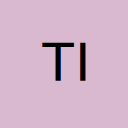# Copying multiple sheets to new workbook, problem with array formulas

• I refer to a now closed thread from 2011 that was answered very neatly by Trebor76.

I have modified the macro slightly – see below – however, when copying array formulas, e.g. {SalesAnnually}, instead of converting the array formula to a value, it copies the formula itself.

Is there a way round this?

Secondly, is it possible to modify the Sheets(Array()) line to pick up worksheets from a list in one of the workbooks, example:

Sheet1 (in cell A1)
Sheet2 (in cell A2)
Sheet5 (in cell A3)

Sheets(Array()) seems unforgiving when referencing cells, e.g. Sheets(Array(A1,A2,A3)).Copy

Any help would be gratefully received – thank you.

• Re: Copying multiple sheets to new workbook, problem with array formulas

Hello,

Quote from tired&emotional;800684
Code
1. For Each wst In Worksheets
2. With Cells
3. .Copy
4. .PasteSpecial xlPasteValues
5. End With
6. Range("A1").Select
7. Next wst

try this one:

Code
1. For Each wst In ActiveWorkbook.Worksheets
2. With wst
3. .Cells.Copy
4. .Cells.PasteSpecial xlPasteValues
5. Application.Goto .Range("A1")
6. End With
7. Next wst
8. Application.CutCopyMode = False

And for the second question regarding Sheets(Array()) try this:

Code
1. sheets(array(range("A1").Value, range("A2").Value, range("A3").value)).copy

Hope it helps.
s.

• Re: Copying multiple sheets to new workbook, problem with array formulas

Rather than using the whole cells, use UsedRange

[vb]For Each wst In ActiveWorkbook.Worksheets
With wst.UsedRange
.Copy
.PasteSpecial xlPasteValues
Application.Goto .Range("A1")
End With
Next wst
Application.CutCopyMode = False[/vb]

• Re: Copying multiple sheets to new workbook, problem with array formulas

sysss

It certain did help, you've answered both my questions - thank you.

However, my workbook contains 70 worksheets.

I allow the user to select as many worksheets as they want to create a new values-only workbook, as with the following example:

Overview (in cell A1)
General Assumptions (in cell A2)
Calculations (in cell A3)
[Left blank] (in cell A4)
.
.
.
[Left blank] (in cell A70)

Each of these cells has a data validation drop-down list of all worksheets within the workbook from which the user can choose (but cells may be left blank).

The issue is I don't know how many worksheets the user will select and therefore the Sheets(Array()) method referencing cells A1 to A70 will be large, and will presumably give blank sheets within the new values-only workbook.

Are you able to help me with this refinement?

Thank you

T&E

• Re: Copying multiple sheets to new workbook, problem with array formulas

Krishnakumar

Thank you for your solution, which I've gone with, as it is slightly faster!

Many thanks

T&E

• Re: Copying multiple sheets to new workbook, problem with array formulas

Hello T&E,

Code
1. ' Declare array
2. Dim varSheetNames
3. ' Populate array with list of sheet names assuming that there is no blank in column A between them and also there are no data below the last one. If so then different approach is needed.
4. ' List of names has to be in activesheet
5. varSheetNames = Application.Transpose(Range("A1:A" & Range("A" & rows.count).End(xlup).Row))
6. Sheets(varSheetNames).Copy

Also note that there should be some kind of error handling. If any of the sheets listed in A column doesn't exist you receive a runtime error after executing line with .copy.
The list has to start from A1 and without blank cells. The rest of the column A below last item in the list has to be empty.

s.

• Re: Copying multiple sheets to new workbook, problem with array formulas

sysss

Thank you again for your help!

Having understood how your code works, I remembered that I'd created a dynamic range name covering the input area in an earlier attempt to solve this problem.

I've therefore combined the two ideas together as follows:

Code
1. varSheetNames = Application.Transpose(Range("DynamicRangeName"))
2. Sheets(varSheetNames).Copy

I've dealt with the blanks issue by asking the user to avoid them and will add error handling for non-existant sheets as you suggest.

Many thanks

T&E